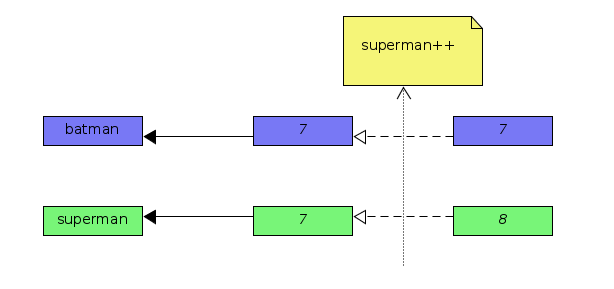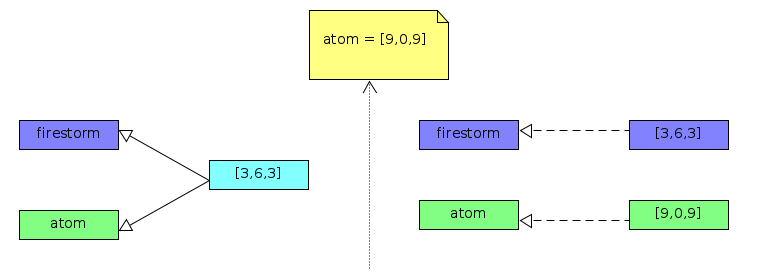# Quick Tip: How JavaScript References Work

By Naveen Karippai
JavaScript
Share:

TL;DR: There are NO pointers in JavaScript and references work differently from what we would normally see in most other popular programming languages. In JavaScript, it’s just NOT possible to have a reference from one variable to another variable. And, only compound values (e.g.. Object or Array) can be assigned by reference.The following terms are used throughout the article:

• scalar – a singe value or unit of data (e.g. integer, boolean, string)
• compound – comprised of multiple values (e.g. array, object, set)
• primitive – a direct value, as opposed to a reference to something that contains the real value.

JavaScript’s scalar types are primitives, but some languages, such as Ruby, have scalar reference types. Note that in JavaScript, the scalar primitive values are immutable while compound values are mutable.

## The Bottom Line on JavaScript References

1. The `typeof` value assigned to a variable decides whether the value is stored with assign-by-value or assign-by-reference.
2. On variable assignment, the scalar primitive values (Number, String, Boolean, undefined, null, Symbol) are assigned-by-value and compound values are assigned-by-reference.
3. The references in JavaScript only point at contained values and NOT at other variables, or references.
4. In JavaScript, scalar primitive values are immutable and compound values are mutable.

## Quick Example of Assign-by-Value:

In the code snippet below, we are assigning a scalar primitive value (a number) to a variable and thus assign-by-value applies here. Firstly, the variable `batman` is initialized and when the variable `superman` is assigned with the value stored in `batman`, it creates a new copy of the value and stores it. When the variable `superman` is modified, `batman` is left unaffected, as they point to distinct values.

``````var batman = 7;
var superman = batman;   //assign-by-value
superman++;
console.log(batman);     //7
console.log(superman);   //8
``````## Quick Example of Assign-by-Reference:

In the code snippet below, we are assigning a compound value (an array) to a variable and thus assign-by-reference applies here. The variables `flash` and `quicksilver` are references to the same value (aka shared value). The references will point to the updated value when the shared value is modified .

``````var flash = [8,8,8];
var quicksilver = flash;   //assign-by-reference
quicksilver.push(0);
console.log(flash);        //[8,8,8,0]
console.log(quicksilver);  //[8,8,8,0]
``````## How to Create a New Reference

When the compound value in a variable is reassigned, a new reference is created. In JavaScript, unlike in most other popular programming languages, the references are pointers to values stored in variables and NOT pointers to other variables, or references.

``````var firestorm = [3,6,3];
var atom = firestorm;   //assign-by-reference
console.log(firestorm); //[3,6,3]
console.log(atom);      //[3,6,3]
atom = [9,0,9];         //value is reassigned (create new reference)
console.log(firestorm); //[3,6,3]
console.log(atom);      //[9,0,9]
``````## How References Work When Values Are Passed as Function Parameters

In the code snippet below, the variable `magneto` is a compound value (an Array), thus it is assigned to variable (function argument) `x` as a reference.

The `Array.prototype.push` method invoked inside the IIFE mutates the value in the variable `magneto` via a JavaScript reference. But, the reassignment of variable `x` creates a new reference and further modifications to it do NOT affect the reference to the variable `magneto`.

``````var magneto = [8,4,8];
(function(x) {        //IIFE
x.push(99);
console.log(x);   //[8,4,8,99]
x = [1,4,1];      //reassign variable (create new reference)
x.push(88);
console.log(x);   //[1,4,1,88]
})(magneto);
console.log(magneto); //[8,4,8,99]
``````

## How to Change the Original Value in a Compound Variable, Passed as a Function Argument via a JavaScript Reference

The solution here would be to modify the existing compound value that the reference is pointing to. In the code snippet below, variable `wolverine` is a compound value (an Array) and, on IIFE invocation, the variable (function argument) `x` is assigned by reference.

The `Array.prototype.length` property can be used to create an empty array by setting its value to `0`. Thus, the variable wolverine is changed to the new value set in variable `x` via a JavaScript reference.

``````var wolverine = [8,7,8];
(function(x) {              //IIFE
x.length = 0;           //make empty array object
x.push(1,4,7,2);
console.log(x);         //[1,4,7,2]
})(wolverine);
console.log(wolverine);     //[1,4,7,2]
``````

## How to Store a Compound Value through Assign-by-Value

The solution here would be to make a manual copy of the compound value and then assign the copied value to a variable. Therefore, the reference of assigned value does NOT point back to the original value.

The recommended approach to create a (shallow) copy of the compound value (Array object) is to invoke `Array.prototype.slice` method on it with no arguments passed.

``````var cisco = [7,4,7];
var zoom = cisco.slice();  //create shallow copy
cisco.push(77,33);
console.log(zoom);         //[7,4,7]
console.log(cisco);        //[7,4,7,77,33]
``````## How to Store a Scalar Primitive Value Through Assign-by-Reference?

The solution here would be to wrap scalar primitive value in a compound value (i.e. an Object or Array) as its property value. Thus, it can be assigned-by-reference. In the code snippet below, scalar primitive value in variable `speed` is set as a property on object `flash`. Therefore, it is assigned-by-reference on IIFE invocation to variable (function argument) `x`.

``````var flash = { speed: 88 };
(function (x) {             //IIFE
x.speed = 55;
})(flash);
console.log(flash.speed);   //55
``````

## SummaryA good understanding of references in JavaScript can help developers to avoid many common mistakes and write better code.

Happy coding!!

## Tags

nilsonjreferencesvariables### Naveen Karippai

JavaScript; Elm; Python Developer